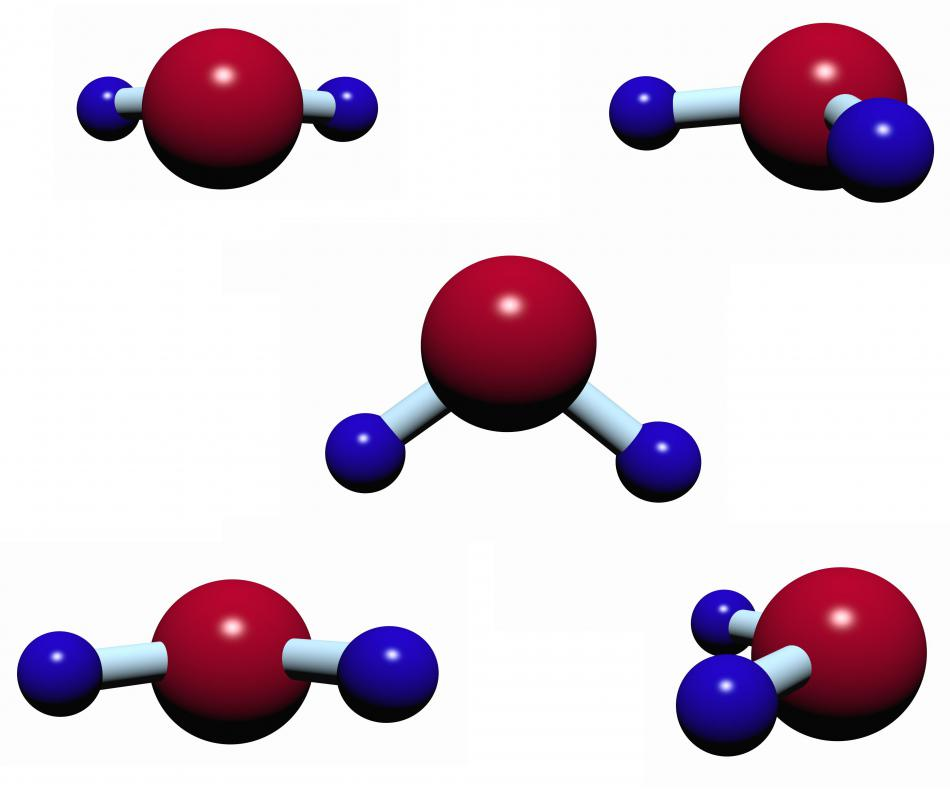# What Is Dissipation Factor?

Andrew Kirmayer

Dissipation factor is a measurement of how inefficient the insulating material of a capacitor is. It typically measures the heat that is lost when an insulator such as a dielectric is exposed to an alternating field of electricity. A capacitor generally has two metal plates and some kind of insulator in between. A ratio of the capacitance when there is an insulating material, to when the plates are separated by air or a vacuum, is often referred to as a dielectric constant. The reciprocal of this ratio defines how the insulating material reacts, and what its resistance is at a particular frequency, yielding a value for the dielectric dissipation factor.Microwaves make use of dissipation factor by polarizing and de-polarizing water molecules.

If a material has low dissipation, this generally means it has a better efficiency. This characteristic is usually defined at a particular frequency. To measure a material’s dissipation, a test with the material between the metal plates is typically done and then a test without it. The results can be expressed in a ratio, which is the dielectric constant typically used to test the dissipation of the material. A dissipation factor test can be completed in other ways, such as using equipment like test cells with different electrode configurations; the method of testing can vary depending on the application.

When a dielectric material is exposed to an electric field, its molecules are reorganized which takes up a significant amount of energy. After the field is removed, the energy cannot be gotten back. Dissipation factor is often referred to as power factor, especially when an alternating current is used with a capacitive circuit not affected by resistance or induced current. A power factor of zero generally indicates that there is no dissipation. Power losses are usually calculated by multiplying the dissipation by the voltage and current.

The dissipation factor of air and a vacuum is typically zero, although air generally does have a loss value small enough to be discounted under most circumstances. This is measured for specific materials, such as polyester for example, at a particular frequency. Wherever a specific material is considered for use in an electrical circuit, it is generally important to understand what its energy loss is like.

There are applications that often make use of dissipation factor, such as the principle involved in microwaving food. The alternating electric fields of a microwave oven cause energy losses from polarizing and de-polarizing water molecules. This results in sufficient heat for food to be cooked.

## You might also Like